Author: Oscar Cronquist Article last updated on June 03, 2023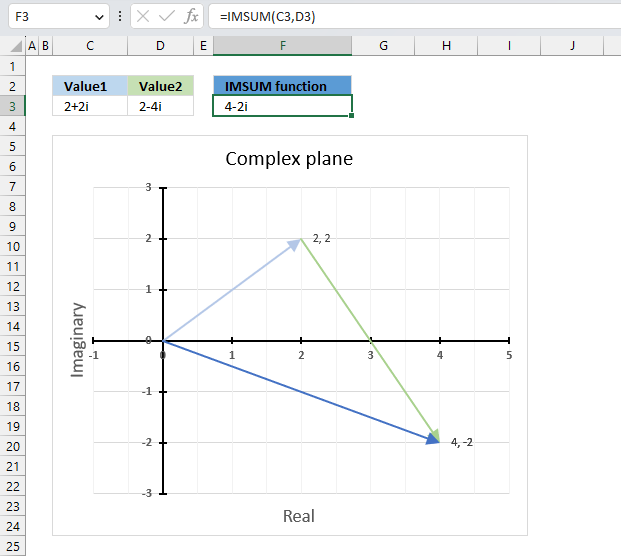The IMSUM function calculates the total of two or more complex numbers in x + yi or x + yj text format.

The letter j is used in electrical engineering to distinguish between the imaginary value and the electric current.

## 1. IMSUM Function Syntax

IMSUM(inumber1, [inumber2], ...)

## 2. IMSUM Function Arguments

 inumber1 Required. A complex number in x+yi or x+yj text format. [inumber2] Optional. A complex number in x+yi or x+yj text format. Up to 255 complex numbers.

## 3. IMSUM function exampleThe image above demonstrates a formula in cell D3 that calculates the total of two complex numbers specified in cells C3 and D3.

Formula in cell D3:

=IMSUM(C3, D3)

### 3.1 Explaining formula

#### Step 1 - Populate arguments

IMSUM(inumber1, inumber2)

becomes

IMSUM(C3, D3)

#### Step 2 - Evaluate the IMSUM function

IMSUM(C3, D3)

becomes

IMSUM("2+2i","2-4i")

and returns

4-2i

## 4. How to calculate the sum of two complex numbers in rectangular form?This example demonstrates how Excel calculates in detail the sum of two complex numbers in rectangular form.

C1 is the first complex number specified in cell C3, C2 is the second complex number specified in cell D3.

C1 = x + yi

C2 = z + wi

To calculate the sum of two complex numbers we need to perform addition to the real and the imaginary numbers separately.

C1+ C2 = (x + yi) + (z + wi) = (x + z) + (y + w)i

C1 = 2+2i

C2 = 2-4i

IMSUM(C1, C2) = C1+ C2 = (2 + 2i) + (2 - 4i) = (2-2) + (2 + (-4))i  = 4-2i

## 5. How to plot the sum of two complex numbers?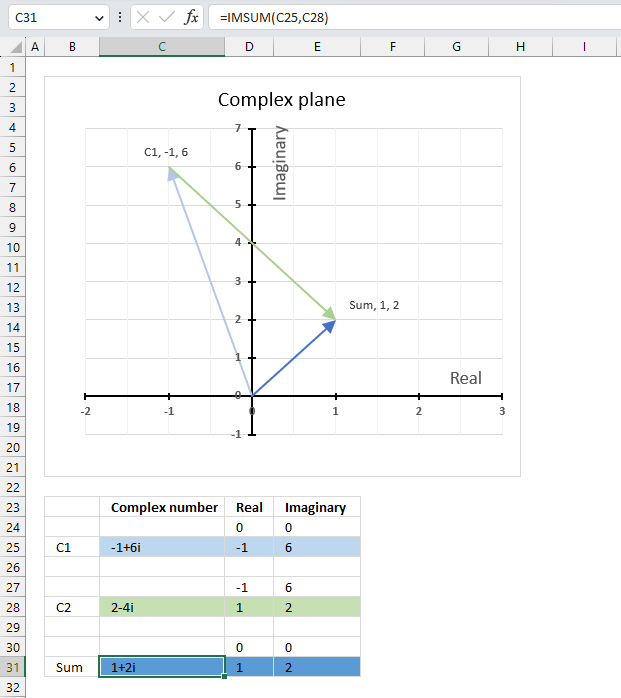The image above shows a scatter chart that displays two different complex numbers and their sum, in total three different complex values.

Complex numbers in rectangular form a+bi have real and imaginary values which are positive, negative or zero, this makes it possible to use a chart with two dimensions x and y.

The imaginary values on the vertical axis (y) and the real values on the horizontal axis (x).

C1 = -1+6i (light blue)

C2 = 2-4i (green)

C1+ C2  = 1+2i (dark blue)

Adding complex numbers means that you can put the second complex number at the first complex number, in other words, the green line starts where the light blue line ends. The dark blue complex number is the sum of C1 and C2 .

### 5.1 Extract real and imaginary numbers from complex numbers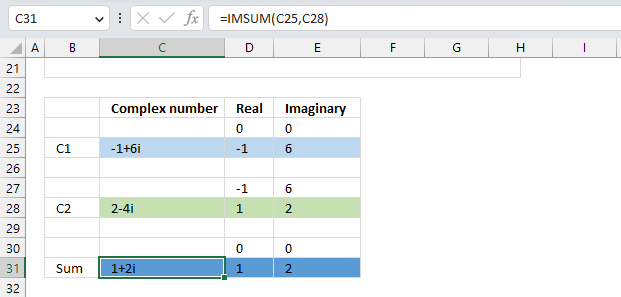The table demonstrated in the image above shows how the data needs to be arranged in order to plot different complex numbers on a chart.

1. Each complex number has a starting point and an ending point.
2. A blank row below each complex number.
3. Formulas for calculating real and imaginary parts.

Formula in cell C31:

=IMSUM(C25,C28)

Formula in cell D25:

=IMREAL(C25)

Formula in cell E25:

=IMAGINARY(C25)

Complex number C2 begins where C1 ends and ends where the sum is located on the complex plane.

### 5.2 Insert scatter chart

1. Select cell range D24:E31.
2. Go to tab "Insert" on the ribbon.
3. Press with mouse on the icon named "Insert Scatter (x,y)", a popup menu appears.
4. Press with left mouse button on the icon "Scatter with Straight Lines and Markers".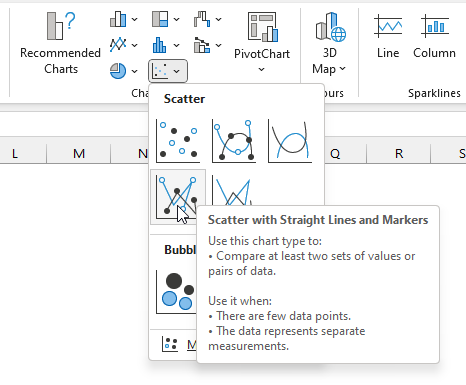5. The chart shows up on the worksheet.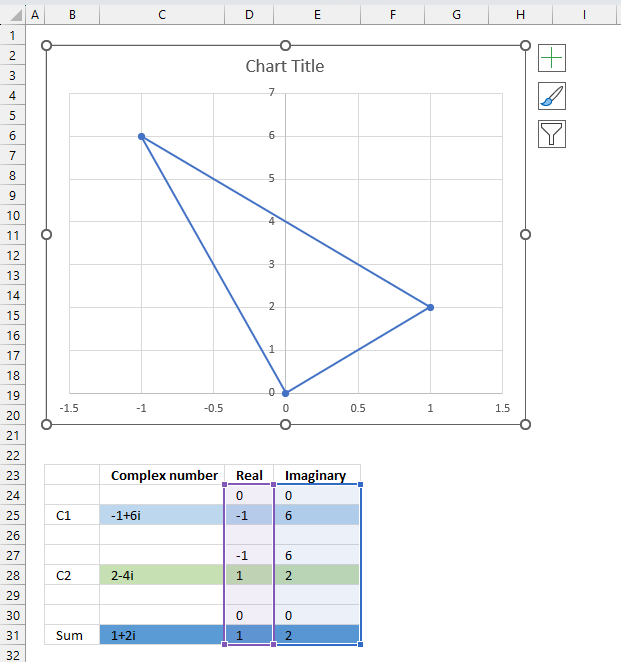Press and hold with left mouse button on the chart border, then drag it to the location you want. Use the "handles" to resize the chart, see the image above.

### 5.3 Create arrows and change colors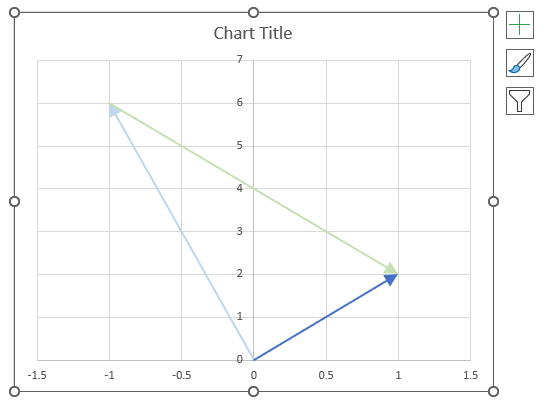1. Double-press with left mouse button on with left mouse button on one of the lines on the chart, all lines will be selected and a settings pane shows up.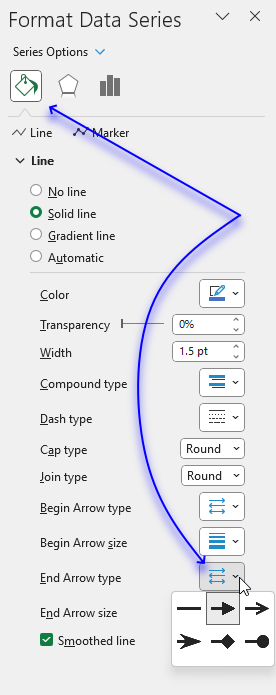2. Press with left mouse button on the "Fill&Line" button.
3. Press with mouse on "End Arrow type", select an arrow.
4. Press with mouse on "End Arrow size, pick a size.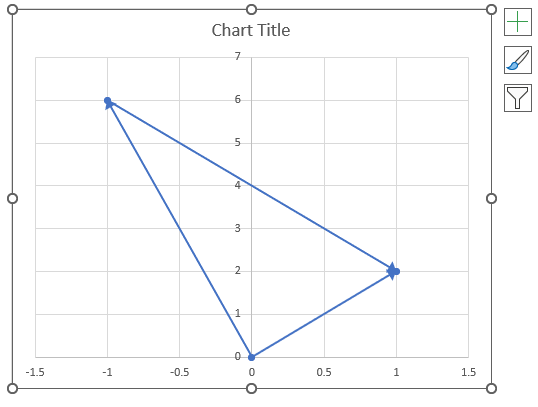1. Press with left mouse button on twice on a line to select a specific line.
2. Press with left mouse button on the color button on the settings pane. A popup menu appears.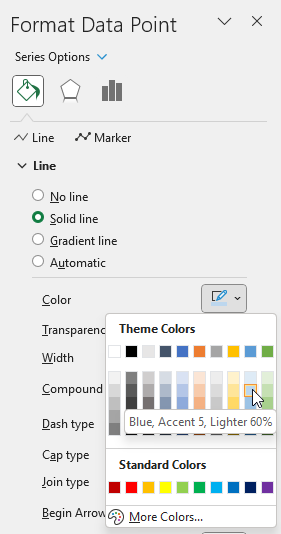3. Pick a color.
4. Repeat steps 1 to 3 with remaining lines.There are still three markers on the chart, here is how to remove them.

1. Select all the lines by press with left mouse button oning on them once.
2. Go to the settings pane.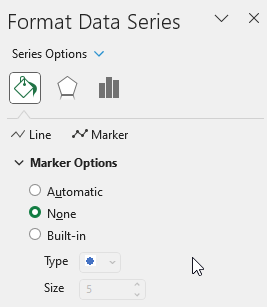3. Press with left mouse button on "Marker Options".
4. Select "None".### 5.4 Change axis min and max value

1. Double-press with left mouse button on one of the y-axis to open the settings pane.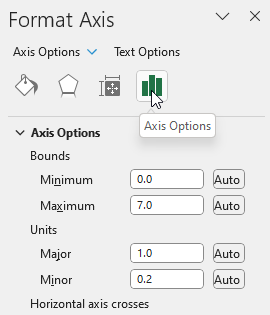2. Press with left mouse button on the "Axis Options" button.
3. Change the Bounds and the Units.
4. Repeat step 1 to 3 with the x-axis.

### 5.5 Change axis markers and line width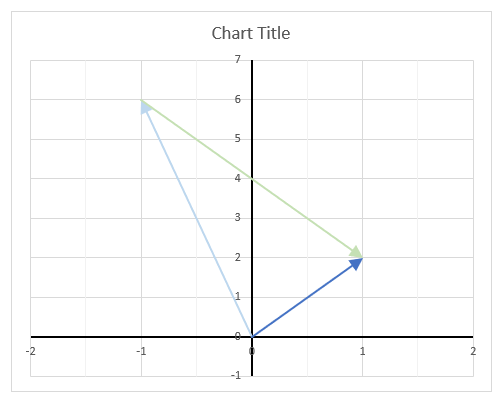1. Select the y-axis.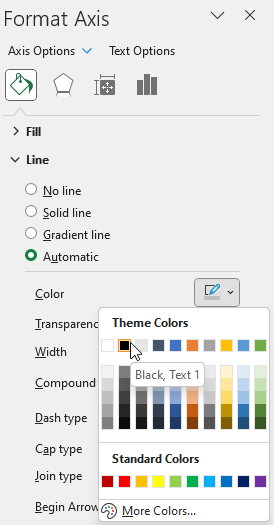2. Press with left mouse button on the "Fill & Line" button.
3. Press with left mouse button on the "Line".
4. Press with left mouse button on the "Color" button. Press with mouse on black.
5. Change the width to 1.51. Press with right mouse button on on one of the lines, a popup menu appears.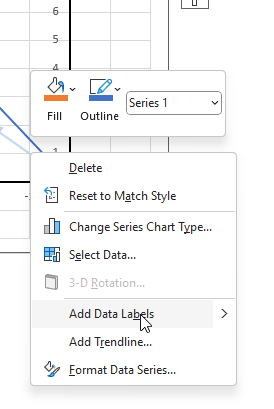2. Press with mouse on "Add Data Labels".
3. Double-press with left mouse button on one of the data labels, the settings pane shows up.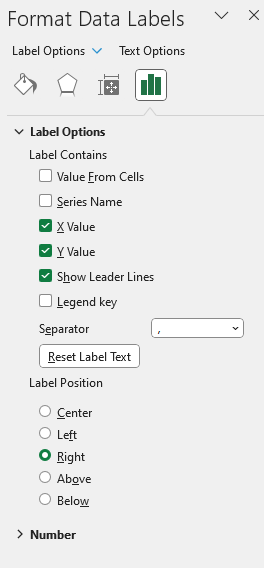4. Select "X Value" as well.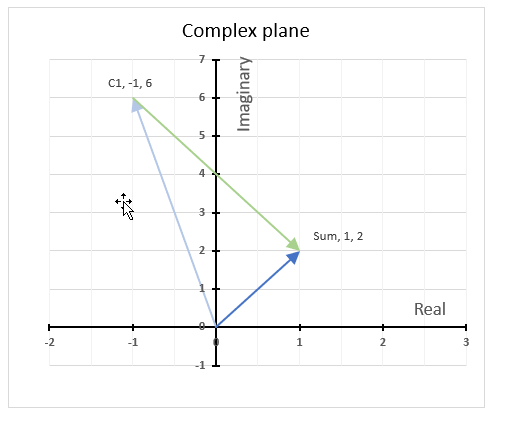Change the chart title and add axis titles.

## 6. How to calculate the midpoint of two complex numbers?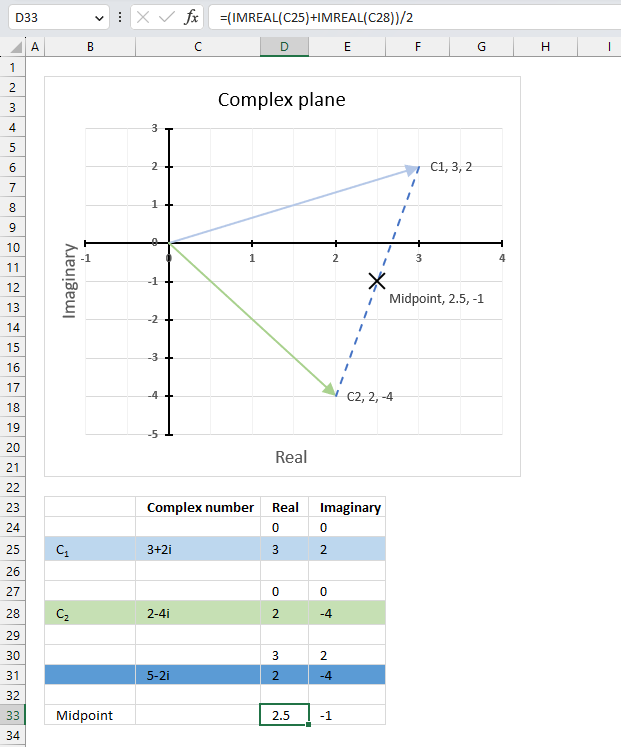The midpoint of the line between two complex numbers x + yi and z + wi is the average of the real and imaginary numbers at the endpoints.

C1 = x + yi

C2 = z + wi

To calculate the average we need to add the real and imaginary numbers separately and then divide by 2.

Midpoint: (x+z) / 2 + ((y+w) / 2)i

Excel formula in cell D33:

=(IMREAL(C25)+IMREAL(C28))/2

Excel formula in cell E33:

=(IMAGINARY(C25)+IMAGINARY(C28))/2

### Explaining formula in cell D33

#### Step 1 - Calculate real number

The IMREAL function calculates the real coefficient of a complex number in x + yi or x + yj text format.

Function syntax: IMREAL(inumber)

IMREAL(C25)

becomes

IMREAL("3+2i")

and returns 3.

#### Step 2 - Add real numbers

The plus sign lets you add numbers in an Excel formula.

IMREAL(C25)+IMREAL(C28)

becomes

3+2

equals 5.

#### Step 3 - Divide sum by 2

The division operator lets you divide numbers in an Excel formula.

(IMREAL(C25)+IMREAL(C28))/2

becomes

5/2

equals 2.5